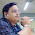### Man Ladder Bottle Puzzle - Knowledge Factory Puzzle

Explanation
Equation 1: (7+3) + (7+3) + (7+3) = 30 (Man = 7 & Glass Bottle = 3 from equation 3)
Equation 2: 10 + 5 + 5 = 20 (Ladder = 5)
Equation 3: 5 + 3 + 3 = 11
Equation 4: 7 + (3 x 4) = 19 (Man = 7; Glass Bottle = 3 & Ladder = 4 as it has only 4 leg holders in last equation)

1.I don't understand last equation

2.forth equation will be - 7 + 3 * 5 = 22

1.Last 5 is wrong ladder legs are 4

3.The ladder in last line has 4 bars so value is 4
Rest all has 5 bars so value is 5

4.5.6.The glass bottle showcase is exceptionally combined with local players commanding in their particular nations. 750ml empty glass bottles with screw caps

7.Do you realize how to get your cash's value? Here are a few connects to give you the direction of spots from where you can get great quality minimal mammoth stepping stools. Frederick McHale

8.9.